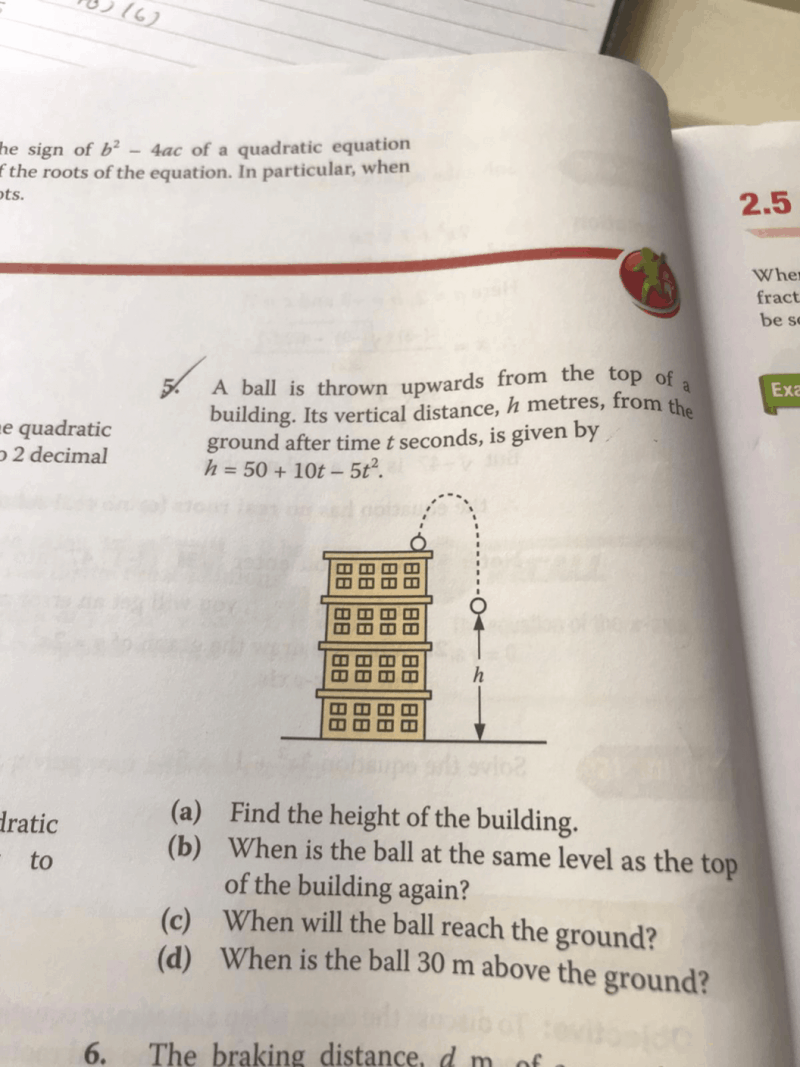# QuestionHi. Any Shifu can help me out with this please? Thank u in advance.

a) The equation h = 50 + 10t – 5t2 essentially models the height of the ball above ground as time passes.

So when t = 0, that would be when the ball is on the roof before it is thrown (assuming the height of the person throwing the ball is negligible)

Substituting t = 0 into the equation,

h = 50 + 10(0) – 5(0)2
h = 50 m

b) Imagine that the ball is thrown upwards then it starts moving downwards, it will reach a level where it is in line with the height where it was thrown before plummeting even further downwards to the ground. This means that the height of the ball above ground should be 50 m.

Substituting h = 50 into the equation,

50 = 50 + 10t – 5t2
5t2 – 10t = 0
t2 – 2t = 0
t(t – 2) = 0
t = 0 or t = 2

t = 0 refers to the time when the ball was at the roof before it was thrown. Therefore the answer is t = 2 seconds after it was thrown, which was when the ball reached the same level from where it was thrown when coming down.

c) when the ball reaches the ground, this means that the distance of the ball above ground (h) must be zero.

Substituting h = 0 into the equation,

0 = 50 + 10t – 5t2
5t2 – 10t – 50 = 0
t2 – 2t – 10 = 0

Using completing the square method,

(t2 – 2t + 1) – 11 = 0
(t – 1)2 – 11 = 0
(t – 1)2 = 11
t = (√11) + 1 or (-√11) + 1 (N.A.)
t ≈ 5.32 seconds

d) Substituting h = 30 into the equation,

30 = 50 + 10(t) – 5(t)2
5t2 – 10t – 20 = 0
t2 – 2t – 4 = 0
(t – 3)(t + 1) = 0
t = 3 seconds or t = -1 seconds (N.A.)